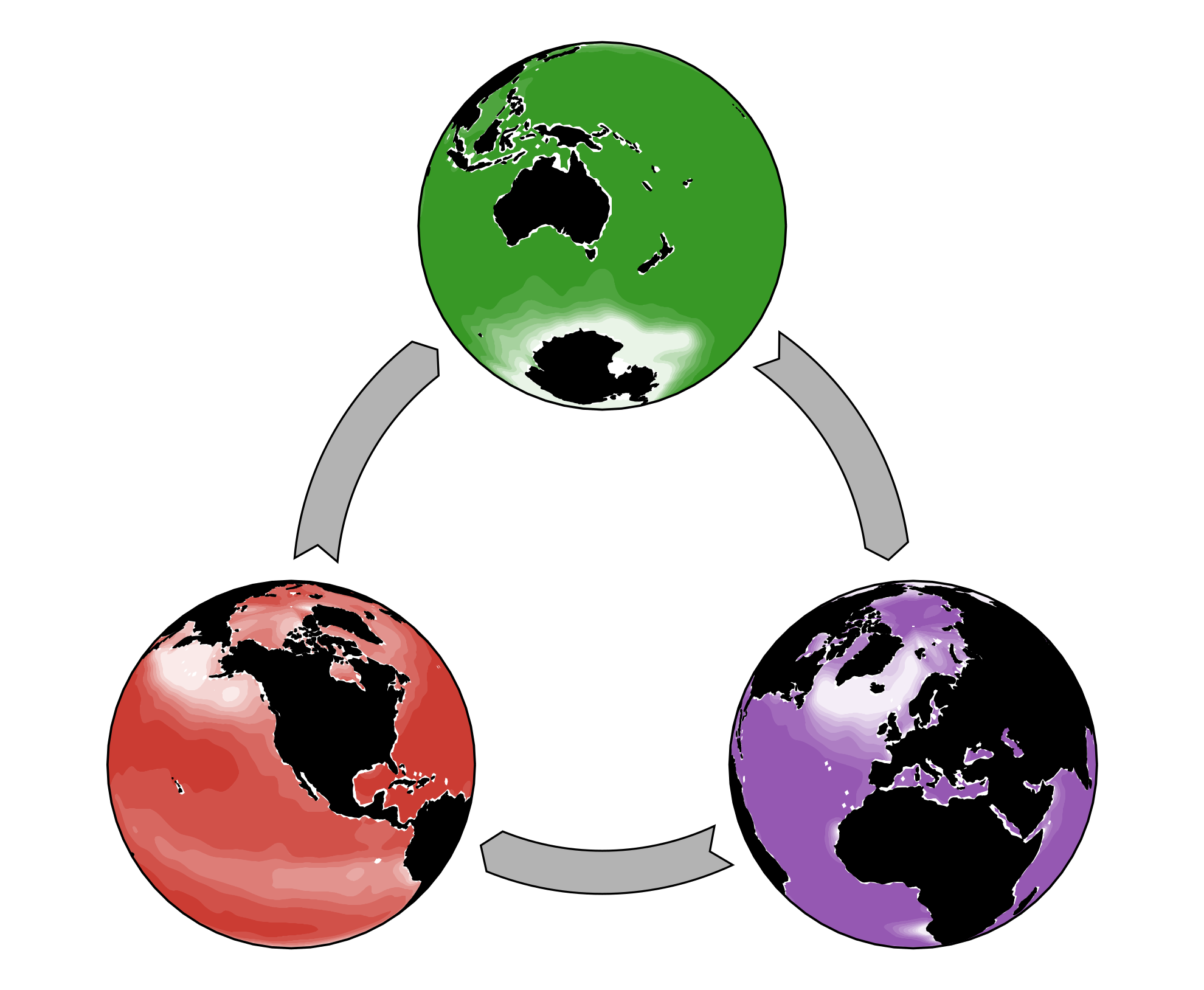# AIBECSAIBECS (for Algebraic Implicit Biogeochemical Elemental Cycling System, pronounced like the cool ibex) is a Julia package that provides ocean biogeochmistry modelers with an easy-to-use interface for creating and running models of the ocean system.

Simply chose some biogeochemical tracers, define their interactions, and select an ocean circulation, and Voilà! — your model is ready to run.

## Getting started

Head over to the documentation and find your way to some simple Jupyter notebooks to get you started with AIBECS!

## The Maths

AIBECS represents global biogeochemical cycles with a discretized system of nonlinear partial differential equations that takes the generic form

$$\frac{\partial \boldsymbol{x}}{\partial t} = \boldsymbol{F}(\boldsymbol{x},\boldsymbol{p})$$

where $\boldsymbol{x}$ is a column vector of the model state variables (i.e., the tracers) and $\boldsymbol{p}$ is a vector of model parameters. (For now, AIBECS only handles steady-state models, for which $\boldsymbol{F}$ does not depend on time.)

This package was developed for models to exploit techniques from linear algebra. A typical example is if the model is linear (affine), i.e., if

$$\boldsymbol{F}(\boldsymbol{x},\boldsymbol{p}) = \mathbf{A} \, \boldsymbol{x} + \boldsymbol{b}$$

In that case, the model’s steady state solution can be computed in a single matrix inversion (via the backslash in MATLAB or Julia), $\boldsymbol{s} = -\mathbf{A}^{-1} \, \boldsymbol{b}$.

However, AIBECS also works for nonlinear problems, i.e., when $\boldsymbol{F}(\boldsymbol{x},\boldsymbol{p})$ is nonlinear, covering a much larger range of models! In this case, AIBECS uses a state-of-the-art Newton-type solver to find the steady-state solution for you, which is much faster than time-stepping the system until it reaches equilibrium. (See, e.g., the work of C. T. Kelley.)

The AIBECS also plays well with the F1Method package, which means that it is ideal for efficient, offline optimization of any biogeochemistry model!##### Benoît Pasquier
###### Research Associate

My research interests include mathematics, oceanography, and computer science.